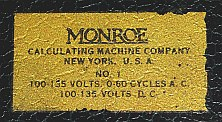previous <<==>> next

 MONROE   Model-1 Have a look at NEWER MODELSHave a look at the Restoration Procedure...
 ``` TECHNICAL DATA of the Electro-Mechanical Calculator MONROE MODEL-1 (OLD) **************************************************************************** Serial Number: 191.823 (engraved inside the carrier-cover) Dimensions: (ca.) Width = 17 " / 43 cm Depth = 13_1/2" / 34 cm Height = 8 " / 20 cm Weight: (ca.) 20_1/2 lbs / 9.5 kg Power Supply: 110 - 135 V AC / DC Mechanics: 10*10 Full-Keyboard Split-Stepped Drum / Geteilte Staffelwalze  10s Carry Mechanism Counter Stepping Mechanism + Animation Functions: Add, Subtract, Multiply, Divide Motorized Carriage Shifting and Division-Automatic! Registers: Input = 10 Decimals Counter (left) = 10 Decimals (NO Carry; Neg.Figures RED) Counter (right) = 10 Decimals (Black Figures with Carry) Arithmetic = 21 Decimals Manufacturer: MONROE Calculating Machine Co. New York / USA 1945H O W T O U S E the (OLD) MONROE MODEL-1 *********************************************** BEFORE STARTING A TASK: ----------------------- UNLOCK the red REPEAT-Button (R) by pressing and pushing it foreward. CLEAR the KEYBOARD by pressing the lower-right red Button (0). UNLOCK RIGHT COUNTER by pressing the button on left-side of the carriage. CLEAR the COUNTER units by pressing down the upper-right handle on carriage. Shift the upper-right red COUNTER-DIRECTION-lever (x) backward. Counter- & arithmetic units are working in the same direction - otherwise, when the lever (:) is shifted foreward, counter- & arithmetic units working opposite. CLEAR the ARITHMETIC unit by lifting the lower-right handle on carriage. (It is intended to press both handles together at the same time.) Bring the CARRIAGE (motorized) to the far left (= Pos.1) by pressing and holding the left SHIFT-KEY on front. ADDITION & SUBTRACTION: ----------------------- Example: 123 + 45 - 6 = 162 ADD: Enter the first number (123) in the far right columns. Press the PLUS-key (+) to transfer the number into arithmetic unit. The counting units display the figure 1. Enter the second number (45). Press the PLUS- key (+) to add the number. The arithmetic unit displays the intermediate sum (168) and the counting units display the figure 2. SUBTRACT: Enter the third number (6). Press the MINUS-key (-). The arithmetic unit displays the result (162) and the counting units are decreased by 1. REMARK: NEGATIVE RESULTS are displayed in the arithmetic unit as the COMPLEMENT of the next higher 10, 100, 1000, ... Example: -12 = 99...9988 MULTIPLICATION: --------------- Example: 123 x 45 = 5535 CLEAR counter and arithmetic units. LOCK the right red REPEAT-Button (R) by pressing and pushing it backward. Enter the multiplicand (123) in the far right columns. The multiplicator (45) has two digits, so the arithmetic unit is moved to position 2 with the right SHIFT-key on front. Repeat pressing the PLUS-key (+), until the first figure of the multiplicator (4) will appear in the 2nd position of the counter units. Move the arithmetic unit to position 1 with the left SHIFT-key on front. Repeat pressing the PLUS-key (+), until the second figure of the multiplicator (5) appears in the 1st position of the counter units. The multiplication is done: The multiplicand (123) stays in the keyboard, the multiplicator (45) is in the counter units and the result (5535) is in the arithmetic unit. DIVISION: --------- Example: 22 : 7 = 3.142857142 Remainder 6 Division requires 3 steps: (A) To Set the Dividend into Arithmetic Unit: ----------------------------------------- For the maximum number of decimals, bring the arithmetic unit to the far right position. Enter the dividend (22) in the far right columns. Press the PLUS-key (+) to transfer into arithmetic unit. (B) To Set the Divisor in the Keyboard: ----------------------------------- CLEAR the counter units by pressing the upper handle on the carriage. Shift the COUNTER-DIRECTION-lever (:) forward Enter the divisor (7) below the dividend (22). LOCK the right red REPEAT-Button (R). (C) To Divide: ---------- Set the upper left red lever forward to divide (:) and the machine will calculate all decimals (and moves the carriage) automatically. The result (3.142857142) is in the counter units, and the remainder (6) is in the arithmetic unit. The divisor (7) stays in the keyboard. R E M A R K S : ============================================================================ Have a look at "Calculating Trickies" ... Have a look at the HOME-MADE 220 VAC POWER PLUG ... impressum: **************************************************************************** © C.HAMANN http://public.BHT-Berlin.de/hamann 04/26/06 ```